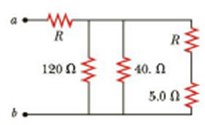Chapter 18, Problem 13P

Chapter
Section
Textbook Problem

The resistance between terminals a and b in Figure P18.13 is 75 Ω. If the resistors labeled R have the same value, determine R.Figure P18.13

To determine
The value of the resistor R.

Explanation

Given Info: The resistors R1=120Ω , R2=40Ω , R3=5.0Ω and unknown resistor R

Explanation:

Formula to calculate the total resistance of the three parallel branches is,

Rp=(1R1+1R2+1R+R3)1

• Rp is the total resistance,
• R1 , R2 and R3 is the resistors,
• R is the unknown resistor,

Substitute 120Ω for R1 , 40Ω for R2 and 5.0Ω for R3 .

Rp=(1120Ω+140Ω+1R+5.0Ω)1=(4120Ω+1R+5.0Ω)1=(130Ω+1R+5.0Ω)1=(30Ω)(R+5

Still sussing out bartleby?

Check out a sample textbook solution.

See a sample solution

The Solution to Your Study Problems

Bartleby provides explanations to thousands of textbook problems written by our experts, many with advanced degrees!

Get Started